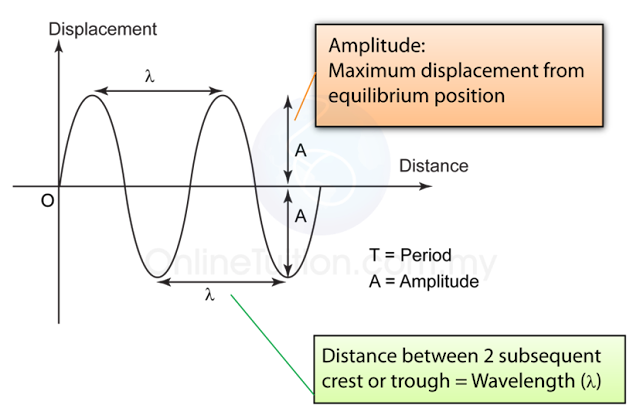# Displacement-Distance Graph

### Displacement – Distance Graph

1. A Displacement – Distance graph shows the position of each particle in a wave relative to its distance from a reference point.
2. The distance between two (2) successive crest or trough is the wavelength.
3. The maximum displacement of the particles from the equilibrium position (displacement = 0) is the amplitude.
4. The amplitude of the wave will increase as the energy transfers by the wave increase and vice versa.

### Comparing Displacement-Time Graph and Displacement- Distance Graph（Displacement-time graph – Graph of oscillation)(Displacement-distance graph – Graph of Waves)

1. Both the displacement-time graph and the displacement distance graph looked similar. However, they are 2 different types of graph.
2. The displacement-time graph illustrates the displacement of an object over time whereas the displacement-distance graph tells the position of the vibrating particles of a wave.
3. For a displacement- time graph, the distance between 2 crest/trough represent the period whereas for the displacement-distance graph, it represents the wavelength.

### 1 thought on “Displacement-Distance Graph”

1.Hi, the time between 2 subsequent crest/trough is called period and the distance between 2 subsequent crest/trough is called wavelength right? I think you typed wrongly because it is stated above that "For a displacement- distance graph, the distance between 2 crest/trough represent the period whereas for the displacement-distance graph, it represents the wavelength." And it should be displacement-time graph. Correct me if Im wrong 🙂